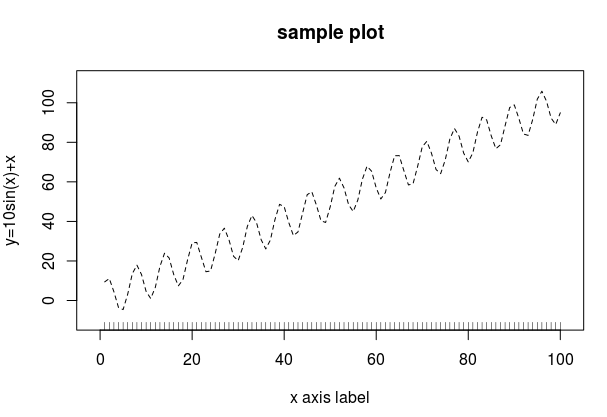# R语言从入门到放弃 (3). 绘图

R关于绘图应该可以写很多, 不过这里只列举在compstat这门课里最经常用的几个函数. 关于R的绘图, 详细了解可以运行 `demo(graphics)`或者 `example("plot")`.

R里面的绘图命令分为两类: 一类是"high-level"的"创建新图片"命令, 运行以后会新画一个图; 另一类则是"low-level"的命令, 不会创建新图片, 而只会在当前图片中修改添加(例如添加线条, 添加点等). 下面分别简单介绍, 最后再介绍其他一些绘图配置的命令.

# configurations/parameters

• `par(mfrow=c(1,2))`: 这个命令是设定画图的布局, 把放置图片的区域分为一行两列, 第一个plot的图片在左边, 第二个在右边.
• `col`: 设定画图(线段/点)的颜色, 可以用数字(col=1, 2, ...)也可以用英文(col="red", "gray", "blue"等)
• `lty`: 设定线段类型, 例如lty=1为实线, lty=2为虚线
• `pch`: 设定点的类型(pch="point character")
• `cex`: 似乎是设定文字大小的, 一般设置cex=0.6

# high level命令: plot

`plot`的内容或者为一系列x和y坐标, 或者为一个formula(见plot.formula). :

• `type`: 指定画线(`type="l"`)或者画点(`type="p"`, 默认值).

`type="n"`的时候表示不画任何东西 — 然后可以用后面介绍的low level命令往里面添加东西.

• `main`: 设定图片的标题

• `xlab`/`ylab`: x/y轴的文字

• `xlim/ylim`: 设定x/y轴的范围, 例如: `xlim=c(0,10)`.

```> extendrange(1:10)
  0.55 10.45
```

```plot(x,　y,　type="l", lty=2,
xlab="x axis label", ylab="y=10sin(x)+x",
main="sample plot",
xlim=c(-1, 101), ylim=extendrange(y)　)
```# low level命令

### points

`points(x, y)`

### lines

`lines(x, y)`

### abline

abline有多种用法:

• `abline(a=a, b=b)`: 指定斜率和截距(y=ax+b)
• `abline(h=y0)`/`abline(v=x0)`: 画水平/垂直的线
• `abline(reg=lm_fit)`: reg为有coeff的对象(如一个线性fit), 画出fitted line

### rug

`rug(x)`的作用是在x轴添加小线段, 位置为参数`x`.

### lengend

`legend("topleft", c("data", "fitted"), lty=1:2)`

### example

```newpts.x <- c(20, 30, 40, 50)
newpts.y <- c(40, 70, 60, 80)
points(newpts.x, newpts.y, pch='x', col="blue")
lines(newpts.x, newpts.y, lty=3, col="red")

abline(h=30, col="gray")
abline(v=50, col="blue", lty=4)

fit <- lm(y~x) # fit to linear model
abline(fit, lty=1)

legend("topleft", c("data", "linear fit", "lines example", "vertical line"),
lty=c(2, 1, 3, 4), col=c(rep("black",3), "blue"))
```